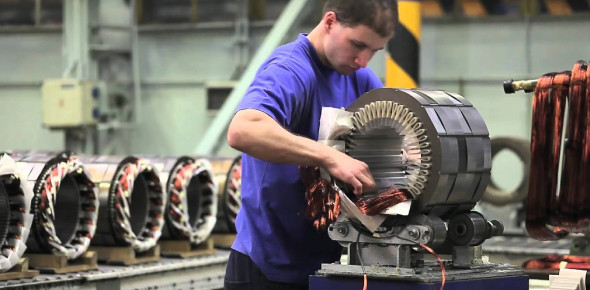# Trivia Questions On Electrical Machines

20 Questions | Total Attempts: 1120Settings.

• 1.
The inductive reactance of a transformer depends on
• A.

Magnetomotive force

• B.

Electromotive force

• C.

Magnetic flux

• 2.
The inductive reactance of a transformer depends on
• A.

Magnetomotive force

• B.

Electromotive force

• C.

Magnetic flux

• 3.
The emf induced in the primary
• A.

Is in phase with the flux

• B.

Lags behind the flux by 90degrees

• C.

• 4.
The flux in transformer core
• A.

• B.

• C.

• 5.
The primary ampereturns are counter balanced by
• A.

Secondary ampere turns

• B.

Primary flux

• C.

Increase in mutual flux

• 6.
Power  transformed from primary to secondary depends upon
• A.

Number of primary turns

• B.

Number of secondary turns

• C.

Magnetic coupling between primary and secondary windings

• 7.
A transformer is supplying pure resistive load.The power factor on primary side will be
• A.

• B.

• C.

Zero

• D.

Unity

• 8.
The voltage regulation of a well designed transformer is of the order of
• A.

20 precentage

• B.

10 percentage

• C.

2 percentage

• D.

Zero

• 9.
The various losses occuring in a power transformer are------ losses
• A.

Copper and iron

• B.

Friction and winding

• C.

Iron,friction and windage losses

• 10.
In the transformer circuit mode,the core loss represented as a
• A.

Series resistance

• B.

Series inductance

• C.

Shunt resistance

• D.

Shunt inductance

• 11.
Smooth cylindrical rotors are usually designed for
• A.

2 or 4 poles

• B.

8 poles

• C.

12 poles

• D.

24 poles

• 12.
A coil consists of
• A.

Two coil-sides

• B.

Two conductors

• C.

Two turns

• D.

One turn

• 13.
The stator of modern alternators are wound for --------- phase groups
• A.

180 degrees

• B.

120 degrees

• C.

60 degrees

• D.

240 degrees

• 14.
Distributed winding is preferred over concentrated winding as it
• A.

Reduces noise

• B.

Reduces the machine size

• C.

Reduces the amount of copper required

• D.

Improves the generated emf wave-form and adds rigidity and mechanical strength to wdg

• 15.
The doubly exicted magnetic systems are
• A.

Solenoids

• B.

Synchronous motors

• C.

Eletromagnetic relays

• 16.
The cooling medium used in large sized synchrounous machines is usually
• A.

Hydrogen

• B.

Air

• C.

Water

• D.

Mineral oil

• E.

Sulphur hexafluoride

• 17.
A polyphase field is
• A.

Pulsating and stationary

• B.

Pulsating and rotating

• C.

Constant in amplitude and roatating at synchronous speed

• D.

Constant in amplitude and sationary in space

• 18.
An alternator with higer value of SCR has.
• A.

Poor voltage regulation and lower stability limit

• B.

Better voltage regulation and higher stability limit

• C.

Poor voltage regulation and higher stability limit

• D.

Better voltage regulation and low stability limit

• 19.
The minimum no of windings required for producing a polyphase field is
• A.

One

• B.

Two

• C.

Three

• D.

None of these

• 20.
Number of slip-rings in a 3-phase synchronous motor will be
• A.

0

• B.

1

• C.

2

• D.

3 or 4

Related TopicsBack to top